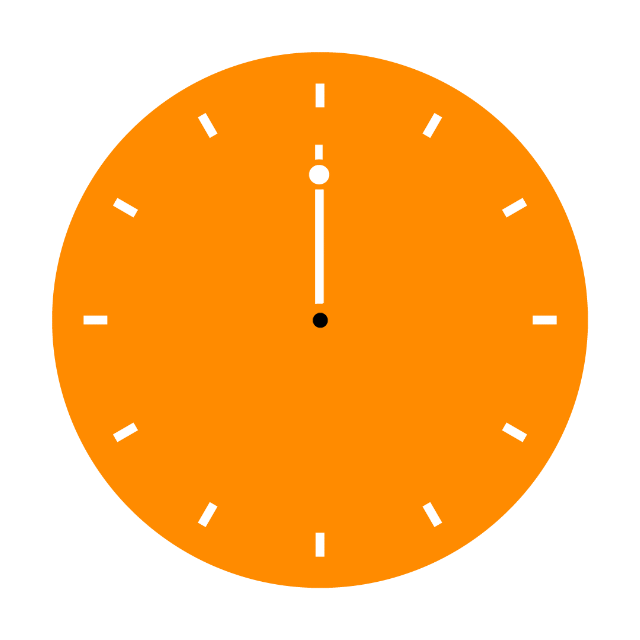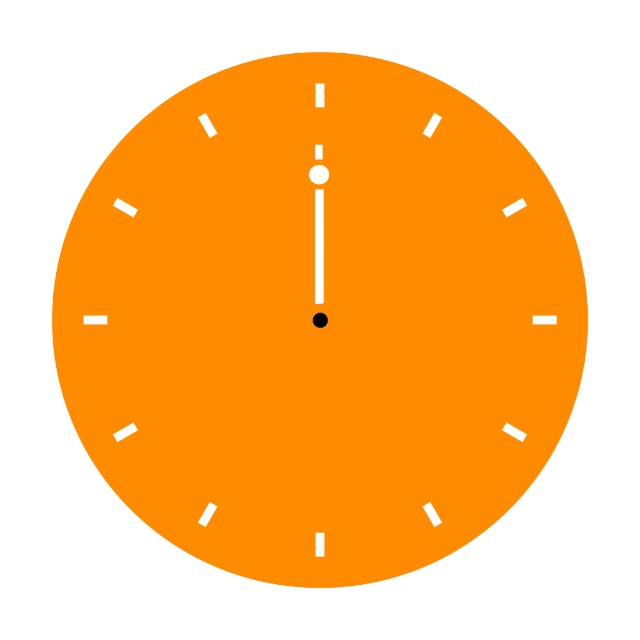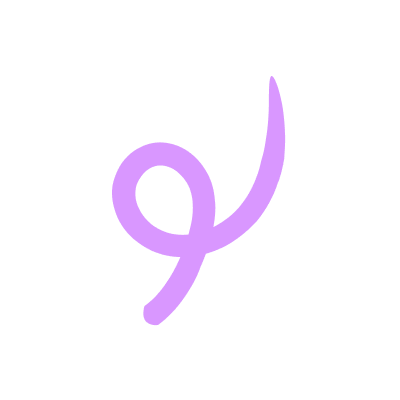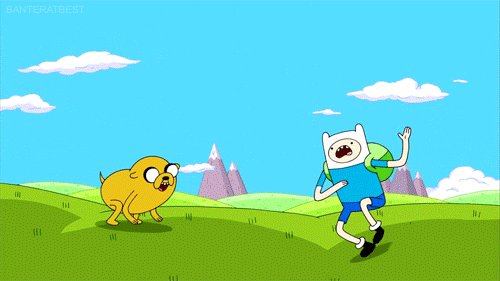IntroductionIntroduction# Rotations

INTRO
A rotation is a type of rigid transformation, which means it changes the position or orientation of an image without changing its size or shape.
A rotation does this by rotating an image a certain amount of degrees either clockwise ↻ or counterclockwise ↺.
For rotations of , , and in either direction around the origin , there are formulas we can use to figure out the new points of an image after it has been rotated.
 Clockwise ↻ Counterclockwise ↺
When we simplify, we can see that the counterclockwise and clockwise rotations are just the reverse of each other. So there are really only 3 rotation formulas we need to remember.
CALCULATOR

KEY STEPS

## How to Perform Rotations

### Step 3. Identify the angle and direction of rotation.

Direction:Angle of Rotation:

### Step 4. Identify the formula that matches the rotation.

When we rotate counterclockwise:
• The axis lines up with the arrow pointing in the negative direction, so the new value is the negative of the old value.
• The axis lines up with the arrow pointing in the positive direction, so the new value is the old value.

### Step 5. Apply the formula to each original point to get the new points.

 Original Point New Point ... ...

### Step 6. Plot the new points.

LESSON
Rotations around the Origin

## Rotations around the Origin

We can use the following formulas for clockwise and counterclockwise rotations of , , and :
 Rotation Formula clockwise or counterclockwise clockwise or counterclockwise clockwise or counterclockwise
Remember clockwise (↻) means the direction in which a clock’s hands would normally rotate, and counterclockwise (↺) means the opposite of that.

### Clockwise ↻### Counterclockwise ↺Once we know which formula we need to use, we can apply the formula to the and values of the original points to get the new points after a rotation around the origin ! 🤩
 Rotation Original New clockwise or counterclockwise clockwise or counterclockwise clockwise or counterclockwise
PRACTICE
Rotations around the Origin

## Practice: Rotations Around the Origin

Question 1 of 5: Rotate the following shape clockwise around the origin.

### Step 1. Identify the center of rotation.

LESSON
Rotations NOT around the Origin

## Rotations NOT around the Origin

Rotations that are not around the origin are a little trickier, but still 100% doable if we take it one step at a time.Let’s walk through some examples to help us remember the rotation formulas!
PRACTICE
Rotations NOT around the Origin

## Practice: Rotations NOT Around the Origin

Question 1 of 5: Rotate the following shape clockwise around the point .

### Step 1. Identify the center of rotation.

CONCLUSION
Nice work, look at you go! Thanks for checking out this lesson ☺️🙏. Where to next?Leave Feedback